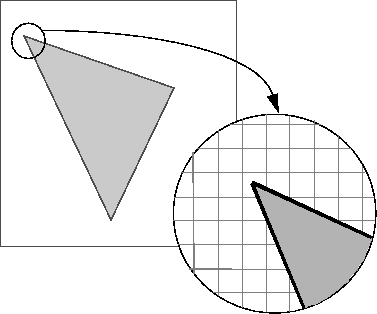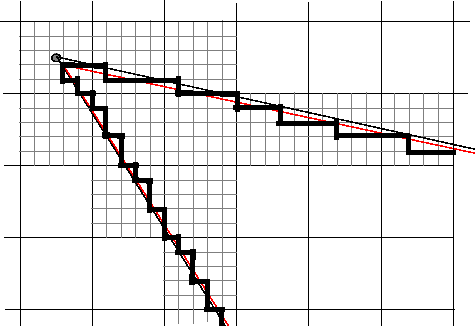## Classify Pixels That Are Partially Enclosed by ROI

When creating a binary mask from a region of interest (ROI), an algorithm must determine which pixels are included in the region. This determination can be difficult when pixels on the edge of a region are only partially covered by the border line.

This figure illustrates a triangular region of interest, examining in close-up one of the vertices of the ROI. The figure shows how pixels can be partially covered by the border of a ROI.To determine which pixels are in the region, many Image Processing Toolbox™ functions use this algorithm:

1. Divide each pixel into a 5-by-5 subpixel grid

The figure shows the pixel that contains the vertex of an ROI, and the 5-by-5 subpixel grid dividing the pixel2. Adjust the position of the vertices

Move each vertex of the polygon to the nearest intersection of the subpixel grid. Round x and y coordinates to the nearest subpixel grid corner. This creates a second, modified polygon.

The figure shows the modified vertex with a red "X".3. Draw a path between adjusted vertices

Form a path from each adjusted vertex to the next, following the edges of the subpixel grid. The figure shows a portion of this modified polygon by the thick dark lines.4. Determine which border pixels are inside the polygon

Use the following rule to determine which border pixels are inside the polygon: if the pixel's central subpixel is inside the boundaries defined by the path between adjusted vertices, then the pixel is inside the region.

In the following figure, the central subpixels of pixels on the ROI border are shaded a dark gray color. Pixels inside the polygon are shaded a lighter gray. Note that the pixel containing the vertex is not part of the ROI because its center pixel is not inside the modified polygon.